# How to Average Only Positive or Negative Numbers of a Range

Suppose both positive numbers and negative numbers exist in a table. If we want to know the average of only positive numbers in this table, we can create a formula to get average of all positive numbers with all negative numbers ignored. In this article, we will help you to construct a formula with AVERAGE and IF functions to get average of only positive numbers or negative numbers.

## EXAMPLE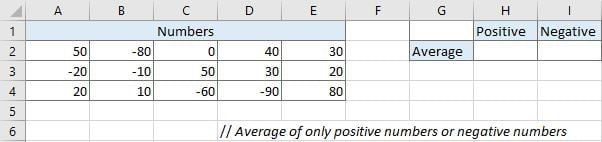Refer to above left side table, we can see both positive numbers and negative numbers are listed in range A2:E4. We want to calculate average of positive numbers and negative numbers separately and save results in H2 and I2 correspondingly.

In this instance, we will enter below formula into H2. We build this formula with AVERAGE and IF function.

`=IF(logical_test,[value_if_true],[value_if_false])`

## STEPS

nctions. The two functions are used frequently in Excel when running mathematics and logical expression.

## FUNCTION INTRODUCTION

a. AVERAGE function returns the average of numbers from a given range reference.

Syntax:

` =AVERAGE(number1, [number2], …)`

b. IF function returns “true value” or “false value” based on the result of provided logical test. It is one of the most popular function in Excel.

Syntax:a. In cell H2, enter the formula =AVERAGE(IF(A2:E4>0,A2:E4,””)). Range reference A2:E4 represents all numbers in this range.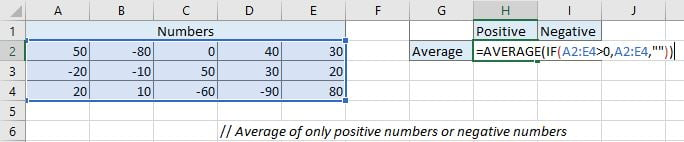This formula will execute IF function firstly to filter and keep all positive numbers in current array. If numbers are greater than 0, they are positive numbers. If logical expression “A2:E4>0” is true (number in A2:E4 is greater than 0), this number will be saved in array A2:E4.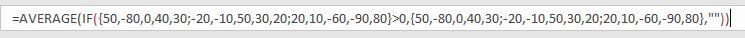After comparing each number in range A2:E4 with 0, below numbers are filtered and saved. Then AVERAGE function will calculate the average of these numbers.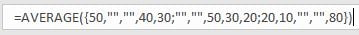b. After entering the formula, press Ctrl + Shift + Enter to load result because this is an array formula. But on Excel 365 you can directly press Enter as usual to load result.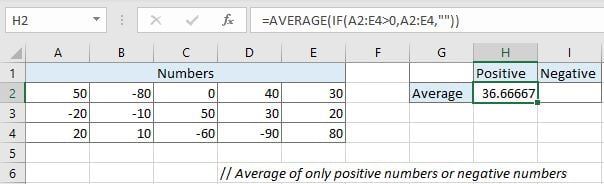c. In I2, enter the formula =AVERAGE(IF(A2:E4<0,A2:E4,””)). Press Ctrl + Shift + Enter to load result.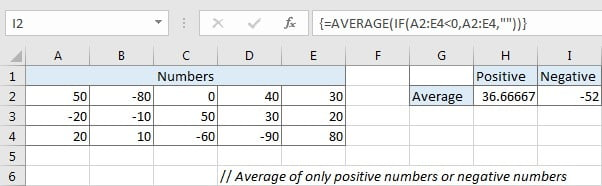### Related Functions

• Excel IF function
The Excel IF function perform a logical test to return one value if the condition is TRUE and return another value if the condition is FALSE. The IF function is a build-in function in Microsoft Excel and it is categorized as a Logical Function.The syntax of the IF function is as below:= IF (condition, [true_value],
• Excel AVERAGE function
The Excel AVERAGE function returns the average of the numbers that you provided.The syntax of the AVERAGE function is as below:=AVERAGE (number1,[number2],…)….
Related Posts

If Cell is This Value or That Value

IF function is frequently used in Excel worksheet to return you expect “true value” or “false value” based on the result of logical test. If you want to see if a cell is A or B, and if one of ...

If Value is Greater Than A Certain Value

IF function is frequently used in Excel worksheet to return you expect “true value” or “false value” based on the logical test result. If you want to see if a value in one cell is greater than a specific value, ...

If Cell is Not Blank

IF function is frequently used in Excel worksheet to return you expect “true value” or “false value” based on the result of created logical test. If you want to see if a cell is blank or not, and leave some ...

If Cell is Blank

IF function is frequently used in Excel worksheet to return you expect “true value” or “false value” based on the result of created logical test. If you want to see if a cell is blank or not, and leave some ...

If Cell Equals Certain Text String

IF function is frequently used in Excel worksheet to return you expect “true value” or “false value” based on the result of created logical test. If you want to see if cell equals a certain text string like “Win”, you ...

If Cell Contains Either Text1 or Text2

IF function is frequently used in Excel worksheet to return “true value” or “false value” based on the logical test result. If you want to see if cell contains certain substring1 like “abc” or substring2 like “def”, and returns true ...

If Cell Contains Certain Text OR Equals Certain Text

IF cell equals certain text IF function is frequently used in Excel worksheet to return “true value” or “false value” based on the logical test result. If you want to test values to see if they equal certain text like ...

Fix #N/A Error For VLOOKUP From Another Sheet

This post will show you how to fix the #N/A error why it occurs when you extract values from another sheet using VLOOKUP function in Excel 2016,2013,2010 or other Excel versions. How can you correct a #N/A error in VLOOKUP ...

VLOOKUP with Two Lookup Tables

VLOOKUP is one of the key functions among all lookup & reference functions in Excel. Today we will show you the application of VLOOKUP function when there are two lookup tables. EXAMPLE Table1 and table2 record the rates of Y2020 ...

Approximate Match with Multiple Criteria by INDEX & MATCH

In Excel, INDEX function and MATCH function are often used together for returning data from specific position. And MATCH function is one of Excel lookup & reference functions that can return approximate value by setting match type. Above all, through ...

Sidebar